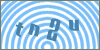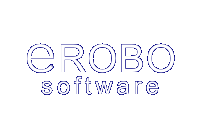Products
ServicesScripts: Cplus :: C++ Arrays :: Library Article #1Developer's SectionCreate an Array in C++
By: Erobo Team Member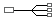Hire a Developer for Related Work / Installation | \$55 hr
 Rating: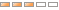| Rate It: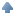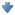Average Votes: (1363) Favorites:Learn to create and populate a C++ array.

In this tutorial we will look at the C++ arrays.

Let's begin by creating a static array. Static arrays are the ones that are not resizable.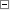Code Snippet 1#include <stdio.h>

//Allocate a static array of 8 slots
//Array of Type int

int main() {

int myArray;

myArray=1892874;
myArray=239583;
myArray=2453;
myArray=256747;
myArray=6834838;
myArray=85954836;
myArray=85737;
myArray=7403853;

}

Dynamic arrays are declared using pointers.Code Snippet 2#include <stdio.h>

//Allocate a dynamic resizable array of 8 slots
//Array of Type int

int main() {

//Declare array using a pointer to memory
int *myArray;

myArray = new int;

myArray=1892874;
myArray=239583;
myArray=2453;
myArray=256747;
myArray=6834838;
myArray=85954836;
myArray=85737;
myArray=7403853;

//resize it

myArray = new int;

//populate

for(int i=0; i< sizeof(myArray); i++) {
myArray[i] = i * 965 -34;
}

}See other Scripts in C++ ArraysSubmit Your Scripts:If you would like to have your C++ scripts published in this section please fill outthe form below:
 [ Refresh Image ]